参考

• matplotlib-绘制精美的图表
• matplotlib.pyplot.plt参数介绍
 1 2 3 4 5 6 7 8 import matplotlib.pyplot as plt labels='frogs','hogs','dogs','logs' sizes=15,20,45,10 colors='yellowgreen','gold','lightskyblue','lightcoral' explode=0,0.1,0,0 plt.pie(sizes,explode=explode,labels=labels,colors=colors,autopct='%1.1f%%',shadow=True,startangle=50) plt.axis('equal') plt.show()

matplotlib图标正常显示中文

 1 2 3 import matplotlib.pyplot as plt plt.rcParams['font.sas-serig']=['SimHei'] #用来正常显示中文标签 plt.rcParams['axes.unicode_minus']=False #用来正常显示负号

matplotlib inline和pylab inline

 1 2 %matplotlib inline #notebook模式下 %pylab inline #ipython模式下

OS X
native/GTK)。对于大部分用户而言，默认的后端就已经够用了。Pylab模式还会向IPython引入一大堆模块和函数以提供一种更接近MATLAB的界面。

matplotlib API包含有三层：

为项目设置matplotlib参数

• 使用参数字典(rcParams)
• 调用matplotlib.rc()命令 通过传入关键字元祖，修改参数

axex: 设置坐标轴边界和表面的颜色、坐标刻度值大小和网格的显示
backend: 设置目标暑促TkAgg和GTKAgg
figure: 控制dpi、边界颜色、图形大小、和子区( subplot)设置
font: 字体集（font family）、字体大小和样式设置
grid: 设置网格颜色和线性
legend: 设置图例和其中的文本的显示
line: 设置线条（颜色、线型、宽度等）和标记
patch:

savefig:

verbose:

xticks和yticks:

线条相关属性标记设置

import matplotlib.pyplot as plt
labels='frogs','hogs','dogs','logs'
sizes=15,20,45,10
colors='yellowgreen','gold','lightskyblue','lightcoral'
explode=0,0.1,0,0
plt.axis('equal')
plt.show()

1、backend_bases.FigureCanvas : 图表的绘制领域
2、backend_bases.Renderer : 知道如何在FigureCanvas上如何绘图
3、artist.Artist : 知道如何使用Renderer在FigureCanvas上绘图

用来该表线条的属性

‘-‘ 实线 ‘:’ 虚线
‘–’ 破折线 ‘None’,’ ‘,’’ 什么都不画
‘-.’ 点划线

matplotlib图标正常显示中文

Axes、Figure。一个figure（绘图面板）可以包含多个Axes（图表），每个Axes都有自己对于的Axis(坐标轴)。

线条标记

‘o’ 圆圈 ‘.’
‘D’ 菱形 ‘s’ 正方形
‘h’ 六边形1 ‘*’ 星号
‘H’ 六边形2 ‘d’ 小菱形
‘_’ 水平线 ‘v’ 一角朝下的三角形
‘8’ 八边形 ‘<’ 一角朝左的三角形
‘p’ 五边形 ‘>’ 一角朝右的三角形
‘,’ 像素 ‘^’ 一角朝上的三角形
‘+’ 加号 ‘\
‘None’,’’,’ ‘ ‘x’ X

Artist的属性描述：颜色

b 蓝色 g 绿色
r 红色 y 黄色
c 青色 k 黑色
m 洋红色 w 白色

• 使用HTML十六进制字符串 color='eeefff' 使用合法的HTML颜色名字（’red’,’chartreuse’等）。
• 也可以传入一个归一化到[0,1]的RGB元祖。 color=(0.3,0.3,0.4)

plt.tilte('Title in a custom color',color='#123456'）

import matplotlib.pyplot as plt
plt.rcParams['font.sas-serig']=['SimHei'] #用来正常显示中文标签
plt.rcParams['axes.unicode_minus']=False #用来正常显示负号

背景色

subplot(111,axisbg=(0.1843,0.3098,0.3098)

matplotlib inline和pylab inline

基础确定坐标范围

• plt.axis([xmin, xmax, ymin, ymax])
上面例子里的axis()命令给定了坐标范围。
• xlim(xmin, xmax)和ylim(ymin, ymax)来调整x,y坐标范围

 1 2 3 4 5 6 7 8 9 10 11 12 13 14 15 16 17 18 %matplotlib inline import numpy as np import matplotlib.pyplot as plt from pylab import * x = np.arange(-5.0, 5.0, 0.02) y1 = np.sin(x) plt.figure(1) plt.subplot(211) plt.plot(x, y1) plt.subplot(212) #设置x轴范围 xlim(-2.5, 2.5) #设置y轴范围 ylim(-1, 1) plt.plot(x, y1)%matplotlib inline #notebook模式下
%pylab inline  #ipython模式下
from sklearn.datasets import load_iris
import matplotlib.pyplot as plt
import pandas as pd

叠加图

 1 2 3 4 5 6 7 8 9 import numpy as np import matplotlib.pyplot as plt # evenly sampled time at 200ms intervals t = np.arange(0., 5., 0.2) # red dashes, blue squares and green triangles plt.plot(t, t, 'r--', t, t**2, 'bs', t, t**3, 'g^') plt.show()plt.figure()

 1 2 3 4 5 6 7 8 9 10 11 12 13 14 import matplotlib.pyplot as plt plt.figure(1) # 第一张图 plt.subplot(211) # 第一张图中的第一张子图 plt.plot([1,2,3]) plt.subplot(212) # 第一张图中的第二张子图 plt.plot([4,5,6]) plt.figure(2) # 第二张图 plt.plot([4,5,6]) # 默认创建子图subplot(111) plt.figure(1) # 切换到figure 1 ; 子图subplot(212)仍旧是当前图 plt.subplot(211) # 令子图subplot(211)成为figure1的当前图 plt.title('Easy as 1,2,3') # 添加subplot 211 的标题figure感觉就是给图像ID，之后可以索引定位到它。

plt.figure(figsize=(10,5))
#设置图形界面的尺寸

df=pd.DataFrame(iris,columns=['a','b','c','d'])
#载入iris数据集以及数据处理

plt.text()添加文字说明

• text()可以在图中的任意位置添加文字，并支持LaTex语法
• xlable(), ylable()用于添加x轴和y轴标签
• title()用于添加图的题目
 1 2 3 4 5 6 7 8 9 10 11 12 13 14 15 16 17 18 19 import numpy as np import matplotlib.pyplot as plt mu, sigma = 100, 15 x = mu + sigma * np.random.randn(10000) # 数据的直方图 n, bins, patches = plt.hist(x, 50, normed=1, facecolor='g', alpha=0.75) plt.xlabel('Smarts') plt.ylabel('Probability') #添加标题 plt.title('Histogram of IQ') #添加文字 plt.text(60, .025, r'$\mu=100,\ \sigma=15$') plt.axis([40, 160, 0, 0.03]) plt.grid(True) plt.show()text中前两个参数感觉应该是文本出现的坐标位置。

plt.annotate()文本注释

y)和插入文本的地方xytext(x, y)。[^1]

 1 2 3 4 5 6 7 8 9 10 11 12 13 14 15 import numpy as np import matplotlib.pyplot as plt ax = plt.subplot(111) t = np.arange(0.0, 5.0, 0.01) s = np.cos(2*np.pi*t) line, = plt.plot(t, s, lw=2) plt.annotate('local max', xy=(2, 1), xytext=(3, 1.5), arrowprops=dict(facecolor='black', shrink=0.05), ) plt.ylim(-2,2) plt.show()[^1]:DataHub-Python

plt.plot(df.a,df.b)
plt.show()

plt.xticks()/plt.yticks()设置轴记号

 1 2 3 4 5 6 7 8 9 10 11 12 13 14 15 16 17 18 19 20 21 22 23 24 25 26 27 28 # 导入 matplotlib 的所有内容（nympy 可以用 np 这个名字来使用） from pylab import * # 创建一个 8 * 6 点（point）的图，并设置分辨率为 80 figure(figsize=(8,6), dpi=80) # 创建一个新的 1 * 1 的子图，接下来的图样绘制在其中的第 1 块（也是唯一的一块） subplot(1,1,1) X = np.linspace(-np.pi, np.pi, 256,endpoint=True) C,S = np.cos(X), np.sin(X) # 绘制余弦曲线，使用蓝色的、连续的、宽度为 1 （像素）的线条 plot(X, C, color="blue", linewidth=1.0, linestyle="-") # 绘制正弦曲线，使用绿色的、连续的、宽度为 1 （像素）的线条 plot(X, S, color="r", lw=4.0, linestyle="-") plt.axis([-4,4,-1.2,1.2]) # 设置轴记号 xticks([-np.pi, -np.pi/2, 0, np.pi/2, np.pi], [r'$-\pi$', r'$-\pi/2$', r'$0$', r'$+\pi/2$', r'$+\pi$']) yticks([-1, 0, +1], [r'$-1$', r'$0$', r'$+1$']) # 在屏幕上显示 show()LaTeX。[^2]

[^2]:Matplotlib

1. 使用参数字典(rcParams)
2. 调用matplotlib.rc()命令通过传入关键字元祖，修改参数

plot的方法默认结果是折线图，show()方法用于打印结果移动脊柱 坐标系

 1 2 3 4 5 6 7 ax = gca() ax.spines['right'].set_color('none') ax.spines['top'].set_color('none') ax.xaxis.set_ticks_position('bottom') ax.spines['bottom'].set_position(('data',0)) ax.yaxis.set_ticks_position('left') ax.spines['left'].set_position(('data',0))

plt.legend()添加图例

 1 2 3 4 plot(X, C, color="blue", linewidth=2.5, linestyle="-", label="cosine") plot(X, S, color="red", linewidth=2.5, linestyle="-", label="sine") legend(loc='upper left')matplotlib.pyplot

 1 2 3 4 5 6 7 8 9 10 11 12 13 14 15 16 17 18 19 20 21 22 23 24 25 # Import necessary packages import pandas as pd %matplotlib inline import matplotlib.pyplot as plt plt.style.use('ggplot') from sklearn import datasets from sklearn import linear_model import numpy as np # Load data boston = datasets.load_boston() yb = boston.target.reshape(-1, 1) Xb = boston['data'][:,5].reshape(-1, 1) # Plot data plt.scatter(Xb,yb) plt.ylabel('value of house /1000 ($)') plt.xlabel('number of rooms') # Create linear regression object regr = linear_model.LinearRegression() # Train the model using the training sets regr.fit( Xb, yb) # Plot outputs plt.scatter(Xb, yb, color='black') plt.plot(Xb, regr.predict(Xb), color='blue', linewidth=3) plt.show()axex: 设置坐标轴边界和表面的颜色、坐标刻度值大小和网格的显示 backend: 设置目标暑促TkAgg和GTKAgg figure: 控制dpi、边界颜色、图形大小、和子区( subplot)设置 font: 字体集（font family）、字体大小和样式设置 grid: 设置网格颜色和线性 legend: 设置图例和其中的文本的显示 line: 设置线条（颜色、线型、宽度等）和标记 patch: 是填充2D空间的图形对象，如多边形和圆。控制线宽、颜色和抗锯齿设置等。 savefig: 可以对保存的图形进行单独设置。例如，设置渲染的文件的背景为白色。 verbose: 设置matplotlib在执行期间信息输出，如silent、helpful、debug和debug-annoying。 xticks和yticks: 为x,y轴的主刻度和次刻度设置颜色、大小、方向，以及标签大小。 标记类型：给特殊点做注释 好吧，又是注释，多个例子参考一下！ 我们希望在 2π/32π/3 的位置给两条函数曲线加上一个注释。首先，我们在对应的函数图像位置上画一个点；然后，向横轴引一条垂线，以虚线标记；最后，写上标签。  1 2 3 4 5 6 7 8 9 10 11 12 13 14 15 16 17 t = 2*np.pi/3 # 作一条垂直于x轴的线段，由数学知识可知，横坐标一致的两个点就在垂直于坐标轴的直线上了。这两个点是起始点。 plot([t,t],[0,np.cos(t)], color ='blue', linewidth=2.5, linestyle="--") scatter([t,],[np.cos(t),], 50, color ='blue') annotate(r'$\sin(\frac{2\pi}{3})=\frac{\sqrt{3}}{2}$', xy=(t, np.sin(t)), xycoords='data', xytext=(+10, +30), textcoords='offset points', fontsize=16, arrowprops=dict(arrowstyle="->", connectionstyle="arc3,rad=.2")) plot([t,t],[0,np.sin(t)], color ='red', linewidth=2.5, linestyle="--") scatter([t,],[np.sin(t),], 50, color ='red') annotate(r'$\cos(\frac{2\pi}{3})=-\frac{1}{2}$', xy=(t, np.cos(t)), xycoords='data', xytext=(-90, -50), textcoords='offset points', fontsize=16, arrowprops=dict(arrowstyle="->", connectionstyle="arc3,rad=.2"))线条相关属性标记设置 颜色类型：plt.subplot() plt.subplot(2,3,1)表示把图标分割成2*3的网格。也可以简写plt.subplot(231)。其中，第一个参数是行数，第二个参数是列数，第三个参数表示图形的标号。 用来该表线条的属性 根据以上的内容，我们修改下图形展示 plt.axes() 我们先来看什么是Figure和Axes对象。在matplotlib中，整个图像为一个Figure对象。在Figure对象中可以包含一个，或者多个Axes对象。每个Axes对象都是一个拥有自己坐标系统的绘图区域。其逻辑关系如下^3)：plt.axes-官方文档 • axes() by itself creates a default full subplot(111) window axis. • axes(rect, axisbg=’w’) where rect = [left, bottom, width, height] in normalized (0, 1) units. axisbg is the background color for the axis, default white. • axes(h) where h is an axes instance makes h the current axis. An Axes instance is returned. rect=[左, 下, 宽, 高] 规定的矩形区域,rect矩形简写，这里的数值都是以figure大小为比例，因此，若是要两个axes并排显示，那么axes的左=axes.左+axes.宽，这样axes才不会和axes重叠。 show code:  1 2 3 4 5 6 7 8 9 10 11 12 13 14 15 16 17 18 19 20 21 22 23 24 25 26 27 28 29 30 31 32 33 34 35 http://matplotlib.org/examples/pylab_examples/axes_demo.html import matplotlib.pyplot as plt import numpy as np # create some data to use for the plot dt = 0.001 t = np.arange(0.0, 10.0, dt) r = np.exp(-t[:1000]/0.05) # impulse response x = np.random.randn(len(t)) s = np.convolve(x, r)[:len(x)]*dt # colored noise # the main axes is subplot(111) by default plt.plot(t, s) plt.axis([0, 1, 1.1*np.amin(s), 2*np.amax(s)]) plt.xlabel('time (s)') plt.ylabel('current (nA)') plt.title('Gaussian colored noise') # this is an inset axes over the main axes a = plt.axes([.65, .6, .2, .2], axisbg='y') n, bins, patches = plt.hist(s, 400, normed=1) plt.title('Probability') plt.xticks([]) plt.yticks([]) # this is another inset axes over the main axes a = plt.axes([0.2, 0.6, .2, .2], axisbg='y') plt.plot(t[:len(r)], r) plt.title('Impulse response') plt.xlim(0, 0.2) plt.xticks([]) plt.yticks([]) plt.show()[^3]:绘图: matplotlib核心剖析 线条风格linestyle或ls 描述 线条风格linestyle或ls 描述 ‘-‘ 实线 ‘:’ 虚线 ‘–’ 破折线 ‘None’,’ ‘,” 什么都不画 ‘-.’ 点划线 plt.plot(df.a,df.b,'b.') plt.show() pyplot.pie参数 • matplotlib.pyplot.pie 线条标记colors颜色 找出matpltlib.pyplot.plot中的colors可以取哪些值？ • so-Named colors in matplotlib • CSDN-matplotlib学习之（四）设置线条颜色、形状  1 2 for name,hex in matplotlib.colors.cnames.iteritems(): print name,hex 打印颜色值和对应的RGB值。 • plt.axis('equal')避免比例压缩为椭圆 标记maker 描述 标记 描述 ‘o’ 圆圈 ‘.’ ‘D’ 菱形 ‘s’ 正方形 ‘h’ 六边形1 ‘*’ 星号 ‘H’ 六边形2 ‘d’ 小菱形 ‘_’ 水平线 ‘v’ 一角朝下的三角形 ‘8′ 八边形 ‘<‘ 一角朝左的三角形 ‘p’ 五边形 ‘>’ 一角朝右的三角形 ‘,’ 像素 ‘^’ 一角朝上的三角形 ‘+’ 加号 ‘\ ‘None’,”,’ ‘ ‘x’ X 结果显示，蓝色散点图，效果好多了。 了解了基本画图实现，matplotlab 画图很多地方和R中的ggplot2 有很高的相似度，它们都是面向对象画图。 autopct • How do I use matplotlib autopct?  1 autopct enables you to display the percent value using Python string formatting. For example, if autopct='%.2f', then for each pie wedge, the format string is '%.2f' and the numerical percent value for that wedge is pct, so the wedge label is set to the string '%.2f'%pct. 颜色 下面来看下标签和坐标轴设置 可以通过调用matplotlib.pyplot.colors()得到matplotlib支持的所有颜色。 调整坐标轴范围可以用两个函数axis([xmin, xmax, ymin, ymax]) 或者xlim(xmin, xmax)和ylim(ymin, ymax) 其中xmin和ymin 代表在各自坐标轴的最小值，相反xmax和ymax 代表在各自坐标轴的最大值。 别名 颜色 别名 颜色 b 蓝色 g 绿色 r 红色 y 黄色 c 青色 k 黑色 m 洋红色 w 白色 还是用上面的例子，看下代码实现。 如果这两种颜色不够用，还可以通过两种其他方式来定义颜色值： plt.axis([0,20,0,10]) plt.xlim(0,20) plt.ylim(0,10) 1. 使用HTML十六进制字符串color='eeefff'使用合法的HTML颜色名字（’red’,’chartreuse’等）。 2. 也可以传入一个归一化到[0,1]的RGB元祖。color=(0.3,0.3,0.4) 两段代码的实现效果是相同的：很多方法可以介绍颜色参数，如title()。 在matplotlab中还提供了一个pylab模块，也有axis、xlim和ylim的函数可以实现类似的功能。 plt.tilte('Title in a custom color',color='#123456'） 接着来看下标题的实现。 背景色 在matplotlib中,主标题使用title函数，x轴和y轴标题用xlabel和ylabel 通过向如matplotlib.pyplot.axes()或者matplotlib.pyplot.subplot()这样的方法提供一个axisbg参数，可以指定坐标这的背景色。 plt.title('iris figure') plt.xlabel('x axis') plt.ylabel('y axis') subplot(111,axisbg=(0.1843,0.3098,0.3098) 实现效果如下：基础 同样可以用pylab模块相同函数实现类似功能。 如果你向plot()指令提供了一维的数组或列表，那么matplotlib将默认它是一系列的y值，并自动为你生成x的值。默认的x向量从0开始并且具有和y同样的长度，因此x的数据是[0,1,2,3]. 接着，来了解下如何在一个图中实现多图、多子图以及在一个图中实现多个不同的线条或形状。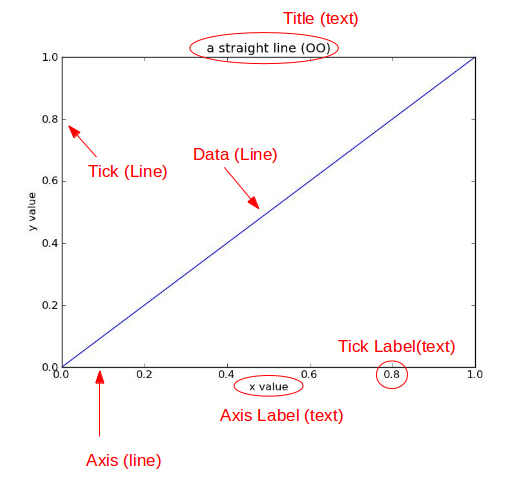绘制多个图用figure函数，绘制子图用subplot函数，多部分则直接在plot中叠加就可以了。 确定坐标范围plt.axis([xmin, xmax, ymin, ymax]) 首先，看下figure实现 上面例子里的axis()命令给定了坐标范围。 plt.figure(1) plt.figure(figsize=(10,5)) plt.plot(df.a,df.b,'b.') plt.figure(2) plt.figure(figsize=(10,5)) plt.plot(df.c,df.d,'g.') plt.show() xlim(xmin, xmax)和ylim(ymin, ymax)来调整x,y坐标范围 实现效果如下 %matplotlib inline import numpy as np import matplotlib.pyplot as plt from pylab import * x = np.arange(-5.0, 5.0, 0.02) y1 = np.sin(x) plt.figure(1) plt.subplot(211) plt.plot(x, y1) plt.subplot(212) #设置x轴范围 xlim(-2.5, 2.5) #设置y轴范围 ylim(-1, 1) plt.plot(x, y1) figure(1)实现效果：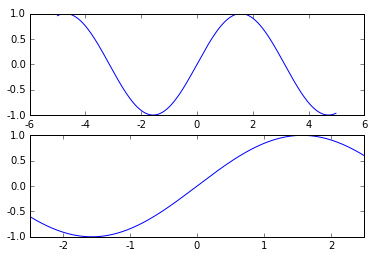figure(2)实现效果：叠加图 同时每个图中的参数需要在各自的figure函数下进行设置。 用一条指令画多条不同格式的线。 下面来了解下子图subplot的实现。 import numpy as np import matplotlib.pyplot as plt # evenly sampled time at 200ms intervals t = np.arange(0., 5., 0.2) # red dashes, blue squares and green triangles plt.plot(t, t, 'r--', t, t**2, 'bs', t, t**3, 'g^') plt.show() subplot(numRows, numCols, plotNum)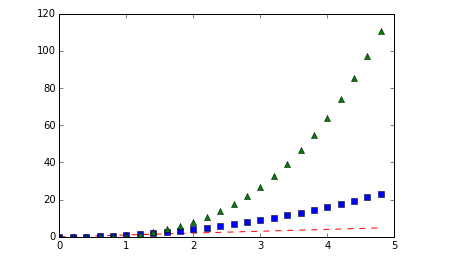图表的整个绘图区域被分成numRows行和numCols列，plotNum参数指定创建的Axes对象所在的区域。 plt.figure() 比如numRows ＝ 2，numCols ＝ 1，就表示整个图区域有两行一列，每一行放一个图。 plotnum=1表示某一幅图放要放在第1行第1列，plotnum=1表示某一幅图放要放在第2行第1列。 你可以多次使用figure命令来产生多个图，其中，图片号按顺序增加。这里，要注意一个概念当前图和当前坐标。所有绘图操作仅对当前图和当前坐标有效。通常，你并不需要考虑这些事，下面的这个例子为大家演示这一细节。 plt.subplot(211) plt.plot(df.a,df.b,'b.') plt.subplot(212) plt.plot(df.c,df.d,'g.') import matplotlib.pyplot as plt plt.figure(1) # 第一张图 plt.subplot(211) # 第一张图中的第一张子图 plt.plot([1,2,3]) plt.subplot(212) # 第一张图中的第二张子图 plt.plot([4,5,6]) plt.figure(2) # 第二张图 plt.plot([4,5,6]) # 默认创建子图subplot(111) plt.figure(1) # 切换到figure 1 ; 子图subplot(212)仍旧是当前图 plt.subplot(211) # 令子图subplot(211)成为figure1的当前图 plt.title('Easy as 1,2,3') # 添加subplot 211 的标题 呈现效果如下：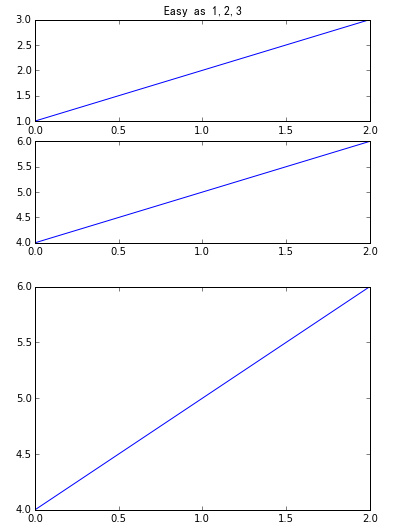subplot中还有其他参数，可以设置整个图的效果，比如axisbg可以为每个子图设置不同的背景色等。 figure感觉就是给图像ID，之后可以索引定位到它。 接着来看下在同一图中画多个部分的实现。 plt.text()添加文字说明 有两种方式可以实现，第一是在一个plot函数中一次性添加多个参数，第二是分成多个plot函数。 text()可以在图中的任意位置添加文字，并支持LaTex语法 #第一种方式 plt.plot(df.a,df.b,'b.',df.c,df.d,'g.') #第二种方式 plt.plot(df.a,df.b,'b.') plt.plot(df.c,df.d,'g.') xlable(), ylable()用于添加x轴和y轴标签 两个代码都能实现如下效果图：title()用于添加图的题目 接着，继续了解图例的设置。 import numpy as np import matplotlib.pyplot as plt mu, sigma = 100, 15 x = mu + sigma * np.random.randn(10000) # 数据的直方图 n, bins, patches = plt.hist(x, 50, normed=1, facecolor='g', alpha=0.75) plt.xlabel('Smarts') plt.ylabel('Probability') #添加标题 plt.title('Histogram of IQ') #添加文字 plt.text(60, .025, r'$\mu=100,\ \sigma=15$') plt.axis([40, 160, 0, 0.03]) plt.grid(True) plt.show() 图例设置使用legend函数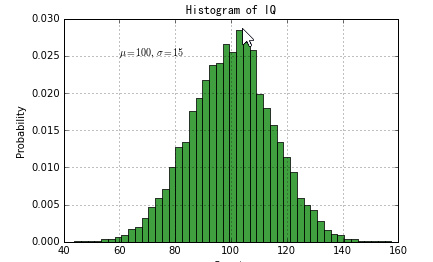这里我们可以取个巧，在画图的时候直接设置标签作为图例的标签，然后通过loc参数设置图例位置，也可以用0-10的整数表示。 还有更复杂的参数，可以参考官网函数详解。 text中前两个参数感觉应该是文本出现的坐标位置。 plt.plot(df.a,df.b,'b.',label='blue') plt.plot(df.c,df.d,'g.',label='green') plt.legend(loc='upper left') plt.annotate()文本注释 显示效果如下：在数据可视化的过程中，图片中的文字经常被用来注释图中的一些特征。使用annotate()方法可以很方便地添加此类注释。在使用annotate时，要考虑两个点的坐标：被注释的地方xy(x, y)和插入文本的地方xytext(x, y)。[^1] 我们还可以对图形进行文本标注。 import numpy as np import matplotlib.pyplot as plt ax = plt.subplot(111) t = np.arange(0.0, 5.0, 0.01) s = np.cos(2*np.pi*t) line, = plt.plot(t, s, lw=2) plt.annotate('local max', xy=(2, 1), xytext=(3, 1.5), arrowprops=dict(facecolor='black', shrink=0.05), ) plt.ylim(-2,2) plt.show() 图形标注可以使用函数text,例如text(1,2,’this is a text’,color=’red’) ,其中1,2表示文本在图中的位置，‘this is a text’是文本内容，其他可以设置字体颜色等。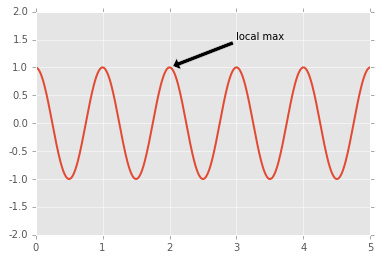plt.plot(df.a,df.b,'b.',label='blue') plt.plot(df.c,df.d,'g.',label='green') plt.text(1,2,'this is a text',color='red') plt.xticks()/plt.yticks()设置轴记号 显示效果如下：现在是明白干嘛用的了，就是人为设置坐标轴的刻度显示的值。 另外还有一种更细致的文本标注方式，用annotate函数 实现，可以用箭头指向文字所标注的位置。 # 导入 matplotlib 的所有内容（nympy 可以用 np 这个名字来使用） from pylab import * # 创建一个 8 * 6 点（point）的图，并设置分辨率为 80 figure(figsize=(8,6), dpi=80) # 创建一个新的 1 * 1 的子图，接下来的图样绘制在其中的第 1 块（也是唯一的一块） subplot(1,1,1) X = np.linspace(-np.pi, np.pi, 256,endpoint=True) C,S = np.cos(X), np.sin(X) # 绘制余弦曲线，使用蓝色的、连续的、宽度为 1 （像素）的线条 plot(X, C, color="blue", linewidth=1.0, linestyle="-") # 绘制正弦曲线，使用绿色的、连续的、宽度为 1 （像素）的线条 plot(X, S, color="r", lw=4.0, linestyle="-") plt.axis([-4,4,-1.2,1.2]) # 设置轴记号 xticks([-np.pi, -np.pi/2, 0, np.pi/2, np.pi], [r'$-\pi$', r'$-\pi/2$', r'$0$', r'$+\pi/2$', r'$+\pi$']) yticks([-1, 0, +1], [r'$-1$', r'$0$', r'$+1$']) # 在屏幕上显示 show() 比如以下代码：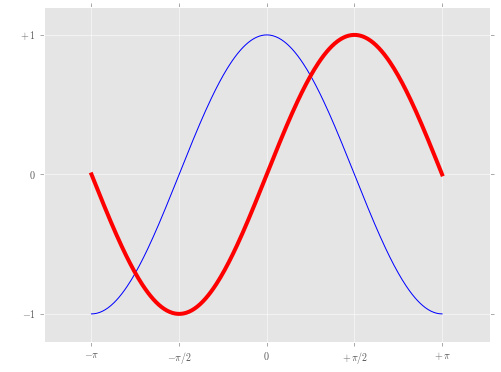plt.plot(df.a,df.b,'b.',label='blue') plt.plot(df.c,df.d,'g.',label='green') plt.annotate('this is text', xy=(5, 4), xytext=(3, 4), arrowprops=dict(facecolor='black', shrink=0.01)) 当我们设置记号的时候，我们可以同时设置记号的标签。注意这里使用了 LaTeX。[^2] 显示效果如下：移动脊柱 坐标系 其中xy为要指向的位置，xytext为文本标注位置，arrowprops设置箭头形式。 ax = gca() ax.spines['right'].set_color('none') ax.spines['top'].set_color('none') ax.xaxis.set_ticks_position('bottom') ax.spines['bottom'].set_position(('data',0)) ax.yaxis.set_ticks_position('left') ax.spines['left'].set_position(('data',0)) 接下来继续了解，坐标轴的刻度设置，可以实现自定义刻度位置以及每个刻度的名称， 这个地方确实没看懂，囧，以后再说吧，感觉就是移动了坐标轴的位置。 使用函数xticks和yticks。 plt.legend()添加图例 比如我把上面图的刻度用以下代码表示后。 plot(X, C, color="blue", linewidth=2.5, linestyle="-", label="cosine") plot(X, S, color="red", linewidth=2.5, linestyle="-", label="sine") legend(loc='upper left') plt.plot(df.a,df.b,'b.',label='blue') plt.plot(df.c,df.d,'g.',label='green') plt.xticks([1,3,5,7],['a','b','c','d']) plt.yticks([1,3,5],['x','y','z'])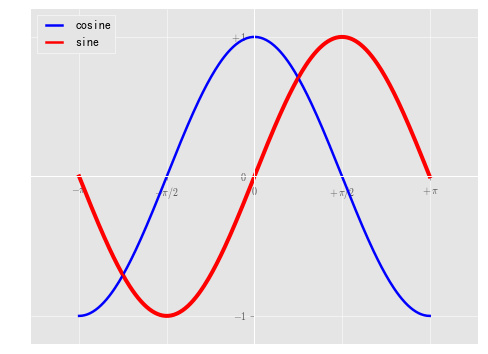结果如下：matplotlib.pyplot 这里我们的刻度更改意义不大，而在可视化过程中有时我们需要对不同的分类变量设置坐标轴时这两个函数就派上用场了。 使用plt.style.use('ggplot')命令，可以作出ggplot风格的图片。 众所周知，在画图方面R的ggplot非常强大，在matplotlib中也能应用ggplot画图风格。 # Import necessary packages import pandas as pd %matplotlib inline import matplotlib.pyplot as plt plt.style.use('ggplot') from sklearn import datasets from sklearn import linear_model import numpy as np # Load data boston = datasets.load_boston() yb = boston.target.reshape(-1, 1) Xb = boston['data'][:,5].reshape(-1, 1) # Plot data plt.scatter(Xb,yb) plt.ylabel('value of house /1000 ($)')
plt.xlabel('number of rooms')
# Create linear regression object
regr = linear_model.LinearRegression()
# Train the model using the training sets
regr.fit( Xb, yb)
# Plot outputs
plt.scatter(Xb, yb, color='black')
plt.plot(Xb, regr.predict(Xb), color='blue',
linewidth=3)
plt.show()
plt.style.use('ggplot')
plt.plot(df.a,df.b,'b.',label='blue')
plt.plot(df.c,df.d,'g.',label='green')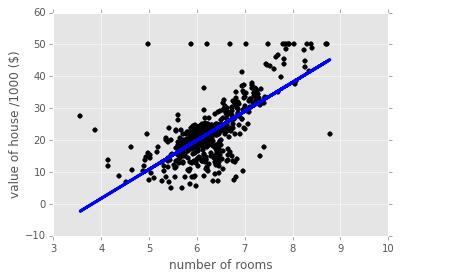savefig('iris.png', dpi = 75）
t = 2*np.pi/3
# 作一条垂直于x轴的线段，由数学知识可知，横坐标一致的两个点就在垂直于坐标轴的直线上了。这两个点是起始点。
plot([t,t],[0,np.cos(t)], color ='blue', linewidth=2.5, linestyle="--")
scatter([t,],[np.cos(t),], 50, color ='blue')

annotate(r'$\sin(\frac{2\pi}{3})=\frac{\sqrt{3}}{2}$',
xy=(t, np.sin(t)), xycoords='data',
xytext=(+10, +30), textcoords='offset points', fontsize=16,

plot([t,t],[0,np.sin(t)], color ='red', linewidth=2.5, linestyle="--")
scatter([t,],[np.sin(t),], 50, color ='red')

annotate(r'$\cos(\frac{2\pi}{3})=-\frac{1}{2}$',
xy=(t, np.cos(t)), xycoords='data',
xytext=(-90, -50), textcoords='offset points', fontsize=16,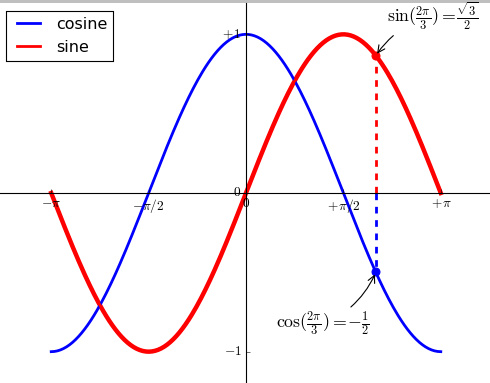plt.subplot()

plt.subplot(2,3,1)表示把图标分割成2*3的网格。也可以简写plt.subplot(231)。其中，第一个参数是行数，第二个参数是列数，第三个参数表示图形的标号。

plt.axes()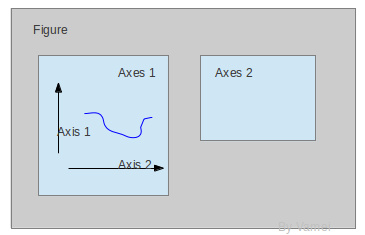plt.axes-官方文档

1. axes() by itself creates a default full subplot(111) window axis.
2. axes(rect, axisbg=’w’) where rect = [left, bottom, width, height]
in normalized (0, 1) units. axisbg is the background color for the
axis, default white.
3. axes(h) where h is an axes instance makes h the current axis. An
Axes instance is returned.

rect=[左, 下, 宽, 高]

show code:

import matplotlib.pyplot as plt
import numpy as np

# create some data to use for the plot
dt = 0.001
t = np.arange(0.0, 10.0, dt)
r = np.exp(-t[:1000]/0.05)        # impulse response
x = np.random.randn(len(t))
s = np.convolve(x, r)[:len(x)]*dt # colored noise

# the main axes is subplot(111) by default
plt.plot(t, s)
plt.axis([0, 1, 1.1*np.amin(s), 2*np.amax(s)])
plt.xlabel('time (s)')
plt.ylabel('current (nA)')
plt.title('Gaussian colored noise')

# this is an inset axes over the main axes
a = plt.axes([.65, .6, .2, .2], axisbg='y')
n, bins, patches = plt.hist(s, 400, normed=1)
plt.title('Probability')
plt.xticks([])
plt.yticks([])

# this is another inset axes over the main axes
a = plt.axes([0.2, 0.6, .2, .2], axisbg='y')
plt.plot(t[:len(r)], r)
plt.title('Impulse response')
plt.xlim(0, 0.2)
plt.xticks([])
plt.yticks([])

plt.show()pyplot.pie参数

matplotlib.pyplot.pie

colors颜色

1. so-Named colors in
matplotlib
2. matplotlib学习之设置线条颜色、形状
for name,hex in matplotlib.colors.cnames.iteritems():
print name,hex

plt.axis('equal')避免比例压缩为椭圆

autopct

How do I use matplotlib autopct?

autopct enables you to display the percent value using Python string
formatting. For example, if autopct=’%.2f’, then for each pie wedge,
the format string is ‘%.2f’ and the numerical percent value for that
wedge is pct, so the wedge label is set to the string ‘%.2f’%pct.

您可能感兴趣的文章:

• python绘图库Matplotlib的安装
• python的绘图工具matplotlib使用实例
• 学习python中matplotlib绘图设置坐标轴刻度、文本
• python+matplotlib实现动态绘制图片实例代码（交互式绘图）
• Python利用matplotlib.pyplot绘图时如何设置坐标轴刻度
• Python使用matplotlib绘图无法显示中文问题的解决方法
• Python使用matplotlib简单绘图示例
• Python 绘图库 Matplotlib
入门教程
• python
matplotlib绘图,修改坐标轴刻度为文字的实例
• Python使用matplotlib实现基础绘图功能示例